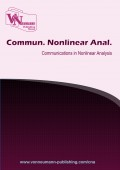# Common coupled fixed point theorem for two pairs of hybrid maps in complex valued metric spaces

Volume 3, Issue 2, pp 52-67### Authors

Konduru Pandu Ranga Rao - Department of Mathematics, Acharya Nagarjuna University, Nagarjuna Nagar -522 510, A.P., India
Shaik Sadik - Department of Mathematics, Sir C R R College of Engineering, Eluru- 534 007, West Godhawari, A.P, India
Saurabh Manro - Department of Mathematics, Thapar University, Patiala, Punjab, India

### Abstract

In this paper, we prove a common coupled fixed point theorem for two hybrid pairs of maps with greatest lower bound property in complex valued metric spaces. We also give an example to illustrate our main theorem.

### Keywords

Complex valued metric, w-compatible maps, hybrid pair, g.l.b property

### References

•  M. Abbas, M. Ali Khan and S. Radenovic, Common coupled fixed point theorems in cone metric spaces for w-compatible mappings, Appl. Math. Comput., 217 (2010), 195–202

•  M. Abbas, M. Arshad and A. Azam, Fixed points of asympotically regular mappings in complex valued metric spaces, Georgian Math. J., 20 (2013), 213–221

•  M. Abbas, Lj. Ciric, B. Damjanovic and M. Ali Khan, Coupled coincidence and common fixed point theorems for hybrid pair of mappings, Fixed Point Theory Appl., 2012 (2012), 11 pages

•  M. Abbas, B. Fisher and T. Nazir, Well-posedness and peroidic point property of mappings satisfying a rational inequality in an ordrerd complex valued-metric spaces, Sci. Stud. Res. Ser. Math. Inform., 22 (2012), 5–24

•  J. Ahmad, C. Klin-Eam and A. Azam, Common fixed points for multi valued mappings in complex valued metric spaces with applications, Abstr. Appl. Anal., 2013 (2013), 12 pages

•  A. Azam, B. Fisher and M. Khan, Common fixed point theorems in complex valued metric spaces, Numer. Funct. Anal. Optim., 32 (2011), 243–253

•  A. Azam, J. Ahmad and P. Kumam, Common fixed point theorems for multi-valued mappings in complex-valued metric spaces, J. Inequal. Appl., 2013 (2013), 12 pages

•  T. G. Bhaskar and V. Lakshmikantham, Fixed point theorems in partially ordered metric spaces and applications, Nonlinear Anal., 65 (2006), 1379–1393

•  S. Chandok and D. Kumar, Some common fixed point results for rational type contraction mappings in complex valued metric spaces, J. Oper., 2013 (2013), 6 pages

•  N. Hussain and A. Alotaibi, Coupled coincidences for multi-valued contractions in partially ordered metric spaces, Fixed Point Theory Appl., 2011 (2011), 15 pages

•  S. M. Kang, M. Kumar, P. Kumar and S. Kumar, Coupled fixed point theorems in complex valued metric spaces, Int. J. Math. Anal., 7 (2013), 2269–2277

•  C. Klin-eam, C. Suanoom, Some common fixed point theorems for generralized contractive type mappings on complex valued metric spaces, Abstr. Appl. Anal., 2013 (2013), 6 pages

•  T. S. Kumar, R. J. Hussain, Common coupled fixed point theorem for contractive type mappings in closed ball of complex valued metric spaces, Adv. Inequal. Appl., 2014 (2014), 1–7

•  M. Kumar, P. Kumar and S. Kumar, Common fixed point theorems in complex valued metric spaces, J. Ana. Num. Theor., 2 (2014), 103–109

•  M. A. Kutbi, J. Ahmad, A. Azam and A. S. Al-Rawashdeh, Generalized common fixed point results via greatest lower bound property, J. Appl. Math., 2014 (2014), 11 pages

•  M. A. Kutbi, A. Azam, J. Ahmad and C. Di Bari, Some common fixed point results for generalized contraction in complex-valued metric spaces, J. Appl. Math., 2013 (2013), 10 pages

•  V. Lakshmikantham, L. Ciric, Coupled fixed point theorems for nonlinear contractions in partially ordered metric spaces, Nonlinear Anal., 70 (2009), 4341–4349

•  H. K. Nashine, M. Imdad and M. Hasan, Common fixed point theorems under rational contractions in complex valued metric spaces, J. Nonlinear Sci. Appl., 7 (2014), 42–50

•  K. P. R. Rao, S. H. Bindu and M. M. Ali, Coupled fixed point theorems in d-Complete topological spaces, J. Nonlinear Sci.Appl., 5 (2012), 186–194

•  K. P. R. Rao, I. Altun and S. H. Bindu, Common coupled fixed point theorems in generalized fuzzy metric spaces, Adv. Fuzzy Syst., 2011 (2011), 6 pages

•  K. P. R. Rao, G. N. V. Kishore and V. C. C. Raju, A coupled fixed point theorem for two pairs of W-compatible maps using altering distance function in partial metric spaces, J. Adv. Res. Pure Math., 4 (2012), 96–114

•  K. P. R. Rao, G. N. V. Kishore and N. V. Luong, A Unique Common Coupled fixed point theorem for four maps under ψ − ϕ contractive condition in partial metric spaces, Cubo, 14 (2012), 115–127

•  K. P. R. Rao, P. R. Swamy and A .Azam, Common coupled fixed point theorems for a pair of hybrid maps in complex valued metric spaces, Adv. fixed point theory, 6 (2016), 277–286

•  K. P. R. Rao, K. R. K. Rao and S. Sedghi, Common coupled fixed point theorems in fuzzy metric spaces, Gazi University Journal of Science, 27 (2014), 739–745

•  F. Rouzkard, M. Imdad, Some common fixed point theorems on complex valued metric spaces, Comput. Math. Appl., 64 (2012), 1866–1874

•  N. Singh, D. Singh, A. Badal and V. Joshi, Fixed point theorems in complex valued metric spaces, J. Egyptian Math. Soc., 24 (2016), 402–409

•  W. Sintunavarat, P. Kumam, Generalized common fixed point theorems in complex valued metric spaces and applications, , 2012 (2012), ., J. Inequal. appl., 2012 (2012), 11 pages

•  K. Sitthikul, S. Saejung, Some fixed points in complex valued metric spaces, Fixed Point Theory Appl., 2012 (2012), 11 pages

•  R. K. Verma, H. K. Pathak, Common fixed point theorems for a pair of mappings in complex valued metric spaces, J. Math. Computer Sci., 6 (2013), 18–26